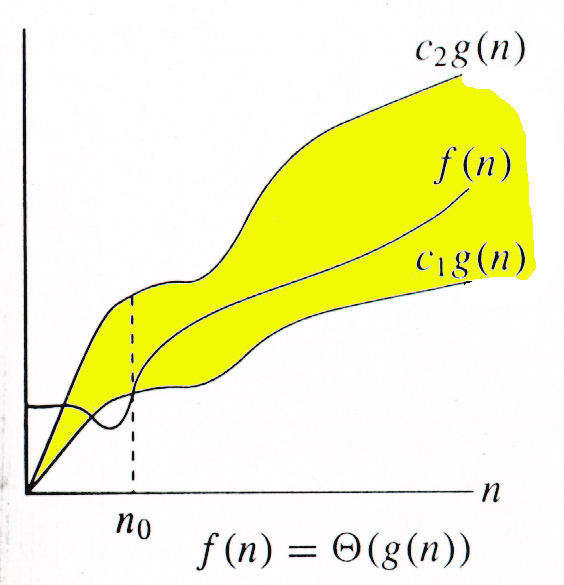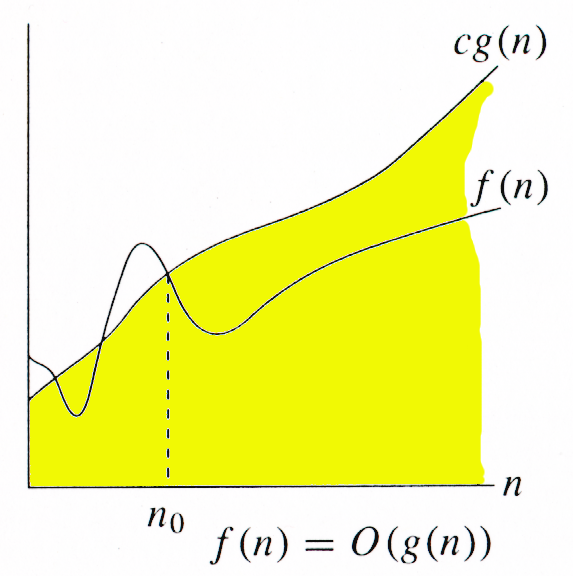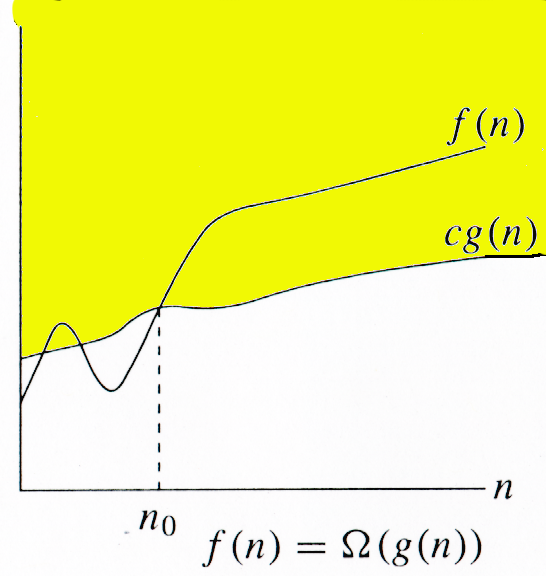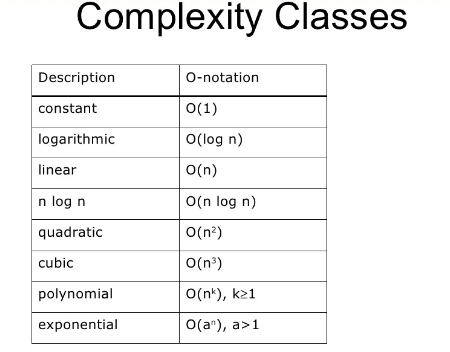### Design & Analysis of Algorithm

Algorithm and Complexity:

What is an Algorithm?

• An algorithm is a step-by-step procedure for solving a problem in a finite amount of time.

• Analysis of Algorithms or performance of algorithms is dependent on Program Performance. It is the amount of computer memory and time needed to run a program.

Measuring Program Performance

• How can one algorithm be ‘better’ than the other?

• The above statement is justified using three criterias namely:

• Time taken for execution

• Size of code

• Space utilized while running and compiling

• There are mainly 2 criterias to judge algorithms:-

1. Time Complexity

2. Space Complexity

Time Complexity

• Time Complexity of an algorithm is the amount of CPU time it needs to run a particular  code to its completion.

• Space Complexity of an algorithm is the amount of memory it needs to run to completion.

Space Complexity

• Memory Space S(P) needed by a program P, consists of two components:

1. A Fixed Part: It is needed for instruction space(byte code), simple variable space, constant’s space etc. Denoted by constant lets say ‘c’.

2. A Variable Part: It is dependent on a particular instance of input and output data. We can denote it by ’Sp’(instance).

S(P) = c + Sp(instance) Time needed
Initialize                        ←              t1
for (i = 1; i<=n; i++) {     ←              set up loop  t2
do something     ←             each iteration t4
}
Finish                            ←             t5
The total ‘cost’ is
t1+t2+n∗t4+t5
Instead of actual time, ti can be treated as how many instructions needed
Frequency Counting
for(i=1;i<10;i++)
print()                       ←        this statement will iterate from 0 to 9 i.e. 10 times
for(i=0;i<=10;i++)
print()                      ←        this statement will iterate ‘n+1’ times (here n=10)
for(i=1;i<=n;i++)
print()
for(i=0;i<n;i++)
print()

The Time Complexity

• A cost function for an algorithm indicates how computation time grows when the amount of data increases

• A special term for it - Time Complexity

• There are generally two Complexities dealing with Complexity of an Algorithm: Time Complexity and Space Complexity

• Space Complexity studies the memory usage (but mostly we pay our attention to time complexity). Suppose Algorithm A’s time complexity is linear then A’s computation time is proportional to the size of A’s input data

Asymptotic Notation

• Asymptotic analysis is a mathematical tool to find time or space complexity of an algorithm without implementing it in a programming language. It is a way of describing a major component of the cost of the entire algorithm.
• Asymptotic notation does the analysis of algorithm independent of all such parameters.

Big O Notation

• Let f(n) and g(n) are two nonnegative functions indicating running time of two algorithms. We say, g(n) is upper bound of f (n) if there exist some positive constants c and n₀ such that 0 ≤ f(n) ≤ c . g(n) for all n ≥ n₀. It is denoted as f (n) = O(g(n)).

• The Big O notation, where O stands for ‘order of’, is concerned with what happens for very large values of n.

• The Big O notation defines the upper bound for the algorithm, it means the running time of algorithm cannot be more than its asymptotic upper bound for any random sequence of data.• For function g(n), we define O(g(n)), big-O of n, as the set :
O(g(n)) = {f(n): ∃ positive constants c and n0,

such that ∀n ≥  n0,

• we have 0 ≤  f(n) ≤ cg(n) }

• Intuitively: Set of all functions whose rate of growth is the same as or lower than that of g(n).

• g(n) is an asymptotic upper bound for f(n).

• f(n) = Θ(g(n)) ⇒ f(n) = O(g(n)).

• Θ(g(n))  ⊂ O(g(n)).

Omega Ω-Notation

• Let f(n) and g(n) are two nonnegative functions indicating running time of two algorithms. We say, g(n) is lower bound of function f (n) if there exist some positive constants c and n₀ such that 0 ≤ c . g(n) ≤ f(n) for all n ≥ n₀. It is denoted as f (n) = Ω(g(n)).

• For function g(n), we define Ω(g(n)), big-Omega of n, as the set:

• This notation is denoted by ‘Ω’, and it is pronounced as “Big Omega”.

• Big Omega notation defines a lower bound for the algorithm.• Ω(g(n)) = {f(n): ∃ positive constants c and n0,

such that ∀n ≥  n0,

• we have 0 ≤ cg(n) ≤ f(n)}.

• Intuitively: Set of all functions whose rate of growth is the same as or higher than that of g(n).

• g(n) is an asymptotic lower bound for f(n).

• f(n) = Θ(g(n)) ⇒ f(n) = Ω(g(n)).

• Θ(g(n))  ⊂ Ω(g(n)).

Big Theta Θ-Notation:

• Let f(n) and g(n) are two nonnegative functions indicating running time of two algorithms. We say, g(n) is tight bound of function f (n) if there exist some positive constants c1, c2 and n₀ such that 0 ≤ c1. g(n) ≤ f(n) ≤c2. g(n) for all n ≥ n₀. It is denoted as f (n) = θ(g(n)).

For function g(n), we define Θ(g(n)), big-Theta of n, as the set:

• This notation is denoted by ’θ’ and it is pronounced as Big theta.

• Big theta defines a tight bound for the algorithm.• Θ(g(n)) = {f(n): ∃ positive constants c1, c2, and n0,

such that ∀n ≥  n0,

• we have 0 ≤ c1g(n) ≤  f(n) ≤ c2g(n)

• Intuitively: Set of all functions that have the same rate of growth as g(n).

• g(n) is an asymptotically tight bound for f(n).

Relations Between Θ, O, Ω notation’s-​​​​​​​

Theorem: For any two functions g(n) and f(n), f(n) = Θ(g(n)) iff

f(n) = O(g(n)) and f(n) = Ω(g(n)).

I.e., Θ(g(n)) = O(g(n)) ∩ Ω(g(n))
In practice, asymptotically tight bounds are obtained from asymptotic upper and lower bounds.

Example:
Θ(g(n)) = {f(n): ∃ positive constants c1, c2, and n0,

such that ∀n ≥  n0,  0 ≤ c1g(n) ≤  f(n) ≤ c2g(n)}

10n2 - 3n = Θ(n2)

What constants for n0, c1, and c2 will work?
Make c1 a little smaller than the leading coefficient, and c2 a little bigger.
To compare orders of growth, look at the leading term.

Exercise: Prove that n2/2-3n= Θ(n2)

​​​​​​​